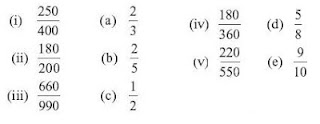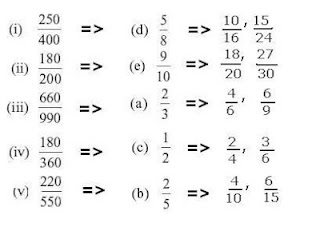## Thursday 29 March 2012

### CBSE Class 6th ( VI) Mathematics Chapter 7. Fractions: Exercise 7.3 Solutions

1. Write the fractions. Are all these fractions equivalent?

Yes, all these fractions are equivalent

No, all these fractions are not equivalent

2. Write the fractions and pair up the equivalent fractions from each row.

3.  Replace in each of the following by the correct number :
 2 8 a. = 7
 5 10 b. = 8
 3 c. = 5 20
 45 15 d. = 60
 18 e. = 24 4

 2 8 a. = 7 28
 5 10 b. = 8 16
 3 12 c. = 5 20
 45 15 d. = 60 20
 18 3 e. = 24 4

4. Find the equivalent fraction of
 5 3
having
(a) denominator 20 (b) numerator 9
(c) denominator 30 (d) numerator 27

 3 12 a. = 5 20
 3 9 b. = 5 15
 3 18 c. = 5 30
 3 27 d. = 5 45

5. Find the equivalent fraction of
 36 48
with
(a) numerator 9 (b) denominator 4

 36 9 a. = 48 12
 36 3 b. = 48 4

6. Check whether the given fractions are equivalent :
(a)

 5 9
 30 54

(b)

 3 10
 12 50

(c)

 7 13

 5 11

(a)
 30 5 = 54 9
Given Fractions are equivalent.

(b)

 12 3 = 50 10
Given Fractions are equivalent

(c)

 5 7 = 11 13
Given Fractions are not equivalent

7. Reduce the following fractions to simplest form :
 48 a. 60
 150 b. 60
 7 c. 28
 84 d. 98
 12 e. 52

 48 4 a. = 60 5
 150 5 b. = 60 2
 7 6 c. = 28 7
 84 3 d. = 98 13
 12 1 e. = 52 4

4 months, Ramesh used up 10 pencils, Sheelu used up 25 pencils and Jamaal
used up 40 pencils. What fraction did each use up? Check if each has used up an
equal fraction of her/his pencils?

The numbers of pencil Ramesh had= 20
The numbers of pencil Sheelu had= 50
The numbers of pencil Jamaal had= 80
After 4 months ...
The numbers of pencil Ramesh had used= 10
The numbers of pencil Sheelu had used= 25
The numbers of pencil Jamaal had used= 40
The numbers of pencil Ramesh has now 20-10= 10
The numbers of pencil Sheelu has now 50-25= 25
The numbers of pencil Jamaal has now 80-40= 40
The fraction of pencil Ramesh used up
 10 20
The fraction of pencil Sheelu used up
 25 50
The fraction of pencil Sheelu used up
 40 80

Dividing both numerator and
denominator by 10 we have
 10 20
=
 1 2
Dividing both numerator and
denominator by 25 we have
 25 50
=
 1 2
Dividing both numerator and
denominator by 40 we have
 40 80
=
 1 2
As all the fractions are equivalent, there for each has used up an equal fraction of her/his pencils
9. Match the equivalent fractions and write two more for each.